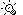# Kilowatts to Calories (Th)/Hour Conversion Calculator

One liter water heted one degree C is 1000 calories.

 Use the following calculator to convert between kilowatts and calories (th)/hour. If you need to convert kilowatts to other units, please try our universal Power Unit Converter.How to use kilowatt to calorie (th)/hour Conversion Calculator Type the value in the box next to "kilowatt [kW]". The result will appear in the box next to "calorie (th)/hour [cal (th)/h]".
 To perform conversions between kilowatt and other Power units please try our Power Unit Converter Convert kilowatt to: watt, exawatt, petawatt, terawatt, gigawatt, megawatt, hectowatt, dekawatt, deciwatt, centiwatt, milliwatt, microwatt, nanowatt, picowatt, femtowatt, attowatt, horsepower, horsepower (550 ft*lbf/s), horsepower (metric), horsepower (boiler), horsepower (electric), horsepower (water), pferdestarke (ps), Btu (IT)/hour, Btu (IT)/minute, Btu (IT)/second, Btu (th)/hour, Btu (th)/minute, Btu (th)/second, MBtu (IT)/hour, MBH, ton (refrigeration), kilocalorie (IT)/hour, kilocalorie (IT)/minute, kilocalorie (IT)/second, kilocalorie (th)/hour, kilocalorie (th)/minute, kilocalorie (th)/second, calorie (IT)/hour, calorie (IT)/minute, calorie (IT)/second, calorie (th)/hour, calorie (th)/minute, calorie (th)/second, foot pound-force/hour, foot pound-force/minute, foot pound-force/second, pound-foot/hour, pound-foot/minute, pound-foot/second, erg/second, kilovolt ampere, volt ampere, newton meter/second, joule/second, exajoule/second, petajoule/second, terajoule/second, gigajoule/second, megajoule/second, kilojoule/second, hectojoule/second, dekajoule/second, decijoule/second, centijoule/second, millijoule/second, microjoule/second, nanojoule/second, picojoule/second, femtojoule/second, attojoule/second, joule/hour, joule/minute, kilojoule/hour, kilojoule/minute

 MBH: